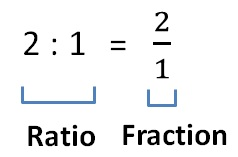Ratios & Fractions are  the same thing.

So, equivalent ratios (link) & Equivalent Fractions  are also the same thing

1. Chapter 12 Class 6 Ratio And Proportion
2. Concept wise
3. Equivalent ratios

Equivalent ratios